# NCERT Solutions for Class 8 Maths Chapter 1 Rational Numbers Ex 1.2

NCERT Solutions for Class 8 Maths Chapter 1 Rational Numbers Ex 1.2 are part of NCERT Solutions for Class 8 Maths. Here we have given NCERT Solutions for Class 8 Maths Chapter 1 Rational Numbers Ex 1.2.

 Board CBSE Textbook NCERT Class Class 8 Subject Maths Chapter Chapter 1 Chapter Name Rational Numbers Exercise Ex 1.2 Number of Questions Solved 7 Category NCERT Solutions

## NCERT Solutions for Class 8 Maths Chapter 1 Rational Numbers Ex 1.2

Question 1.
Represent these numbers on the number line.
(i) $$\frac { 7 }{ 4 }$$
(ii) $$\frac { -5 }{ 6 }$$
Solution.
(i) $$\frac { 7 }{ 4 }$$
We make 7 markings of distance $$\frac { 1 }{ 4 }$$ each on the right of 0 and starting from 0. The seventh marking represents $$\frac { 7 }{ 4 }$$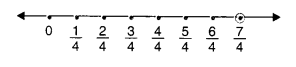(ii) $$\frac { -5 }{ 6 }$$
We make 5 markings of distance $$\frac { 1 }{ 6 }$$ each on the right of 0 and starting from 0. The seventh marking represents $$\frac { -5 }{ 6 }$$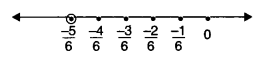Question 2.
Represent $$\frac { -2 }{ 11 } ,\frac { -5 }{ 11 } ,\frac { -9 }{ 11 }$$ on the number line.
Solution.
We make 9 markings of distance $$\frac { 1 }{ 11 }$$ each on the left of 0 and starting from 0.
The second marking represents $$-\frac { 2 }{ 11 }$$ the fifth marking represents $$-\frac { 5 }{ 11 }$$ and the ninth marking represents $$-\frac { 9 }{ 11 }$$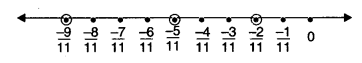Question 3.
Write five rational numbers which are smaller than 2.
Solution.
Five rational numbers which are smaller than 2 are 1, $$\frac { 1 }{ 2 }$$,0, -1, $$-\frac { 1 }{ 2 }$$

Question 4.
Find ten rational numbers between $$-\frac { 2 }{ 5 }$$ and $$\frac { 1 }{ 2 }$$
Solution.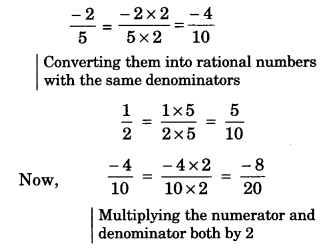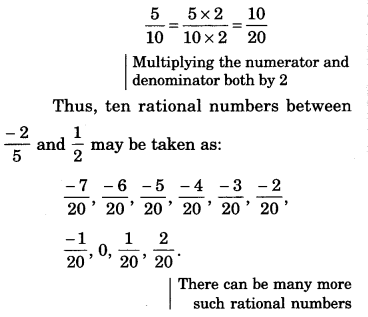Question 5.
Find five rational numbers between: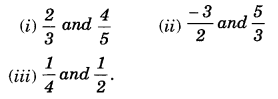Solution.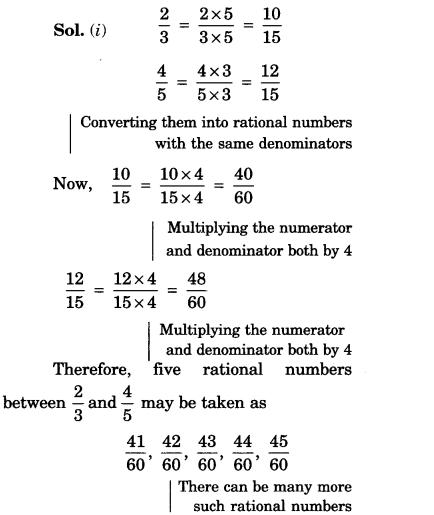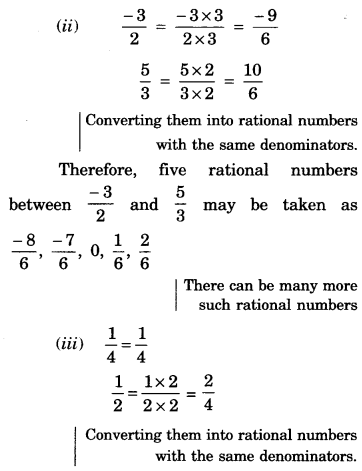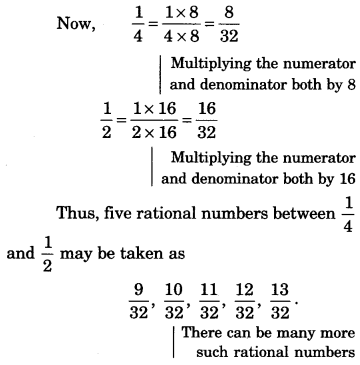Question 6.
Write five rational numbers greater than -2.
Solution.
Five rational numbers greater than -2 are:
$$\frac { -3 }{ 2 }$$, -1, $$\frac { -1 }{ 2 }$$, 0, $$\frac { 1 }{ 2 }$$
There can be many more such rational numbers

Question 7.
Find ten rational numbers between $$\frac { 3 }{ 5 }$$ and $$\frac { 3 }{ 4 }$$
Solution.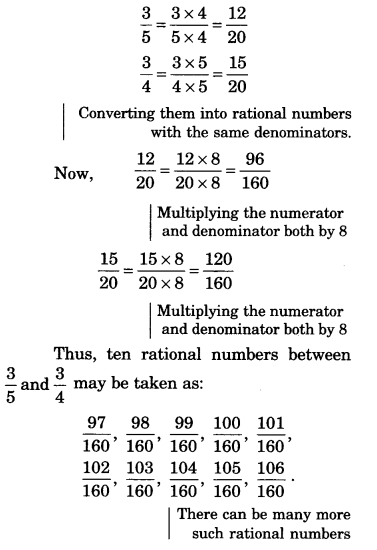We hope the NCERT Solutions for Class 8 Maths Chapter 1 Rational Numbers Ex 1.2 help you. If you have any query regarding NCERT Solutions for Class 8 Maths Chapter 1 Rational Numbers Ex 1.2, drop a comment below and we will get back to you at the earliest.